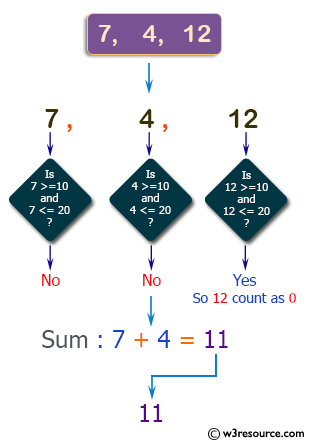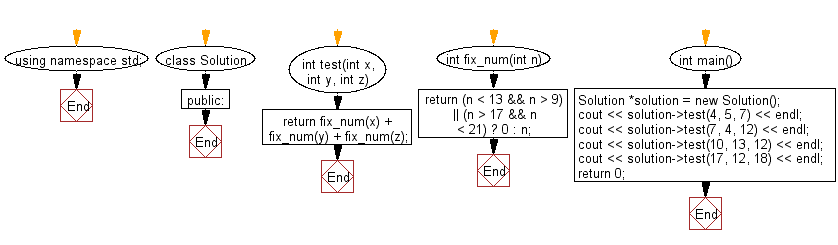﻿ C++ Exercises: Compute the sum of the three given integers - w3resource# C++ Exercises: Compute the sum of the three given integers

## C++ Basic Algorithm: Exercise-54 with Solution

Write a C++ program to compute the sum of the three given integers. However, if any of the values is in the range 10..20 inclusive then that value counts as 0, except 13 and 17.

Sample Solution:

C++ Code :

``````#include <iostream>
using namespace std;
class Solution
{

public:

int test(int x, int y, int z)
{
return fix_num(x) + fix_num(y) + fix_num(z);
}

int fix_num(int n)
{
return (n < 13 && n > 9) || (n > 17 && n < 21) ? 0 : n;
}
};
int main()
{
Solution *solution = new Solution();
cout << solution->test(4, 5, 7) << endl;
cout << solution->test(7, 4, 12) << endl;
cout << solution->test(10, 13, 12) << endl;
cout << solution->test(17, 12, 18) << endl;
return 0;
}
``````

Sample Output:

```16
11
13
17
```

Pictorial Presentation:Flowchart:C++ Code Editor:

Contribute your code and comments through Disqus.

What is the difficulty level of this exercise?

﻿